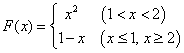FORTRAN五种数据类型中，有两种非数值型数据：逻辑型、字符型。要运用这两种数据，同样要掌握它们的变量说明、常数书写格式、表达式、赋值语句、编辑符、输入输出格式。掌握这两种类型，能实现信息管理方面的强大功能，在处理办公室报表、文件、文本、文本编辑时有足够手段。并使数值型计算也变得更为丰富。

4.2.1 逻辑型数据

a)    逻辑型变量

LOGICAL[(KIND=种别值)][,属性说明表]:: 变量名表[=初始值]

LOGICAL :: L1=.TRUE.,L2

b)    逻辑赋值Program Sample

implicit none

real :: x,y

logical :: in_side

read *, x ; print *,x=,x

in_side=x>1..and.x<2.

if(in_side) then

y=x*x

else

y=1-x

end if

print *,y=,y

End Program Sample

4.2.2 字符型数据

a)    字符变量

CHARACTER[(LEN=整型字符长度表达式[,KIND=种别值])][,属性说明] :: 变量名表[=初始值]

CHARACTER(LEN=12,KIND=1) :: A,B

CHARACTER(KIND=1,LEN=12) :: A,B

CHARACTER(12,1) :: A,B

CHARACTER*12 :: A,B

CHARACTER(LEN=*),PARAMETER :: C_NAME=GIRL

CHARACTER(LEN=*),PARAMETER :: C_NAME=BOY

CHARACTER后面说明的长度是其后所有实体名的公共长度，如果某一变量的长度与其它不同，可以在其变量名后标出自己的特有长度，方法是在变量名后写上*及长度。例加

CHARACTER(LEN=12) :: A,B*5,C,D*7,E

b)    字符子串

A(3:11)    ->  CDE12345F’，

A(I+4:9)   ->  E1234(I=1)，‘1234(I=2)

A(:5)      ->  ABCDE

A(11:)     ->  FGH

A(:)       ->  ABCDE12345FGH

A(3:3)     ->  C

c)    字符操作与赋值

d)    字符比较＞、＜关系操作符＝＝ 关系操作符

NAME==Einstein               ->

NAME==Ein//stein       ->

NAME/=Ein//Stein      ->

NAME==EINSTEIN              ->

e)      字符函数

F90的内部函数中有许多与字符型操作有关的函数供用户使用，可以很方便地进行各种文本编辑。字符与数值相互转换的函数：ICHARCHARIACHRACHAR

F90允许把字符型的值转换成整型值(已知字符，取字符型的序号数值)，也允许把数值型的值转换成字符型的值(已知序号，取它对应的字符)。函数ICHAR的功能是把字符型转换成数值，它的引用方式是：ICHAR(字符实体)。这里自变量的字符实体不一定是单个字符，也可以是字符变量、字符子串、字符数组元素、字符函数值等等以及由它们组成的字符表达式。函数值ICHAR返回字符实体第一个字符的序号值。如果指明要按照ASCII码求它的序号，则本功能函数名应改写IACHAR，例如：

print *, ichar(A), ichar(A_dog), ichar(A_dog//in_my_room)

DO I=0,25

PRINT *,DIMENSION //CHAR(I+6)

END DO求字符串长度的函数：LEN不计尾部空格的字符串长度函数：LEN_TRIM求子串在父串位置的函数：INDEX验证字符串的函数：VERIFY除去尾部空格函数：TRIM比较字符大小的函数：LGELGTLLELLT

 引用方式 含   义 例 LGE(a1,a2) a1是否≥a2 LGE(‘A’，‘B’)值为假 LGT(a1,a2) a1是否＞a2 LGT(‘A’，‘B’)值为假 LLE(a1,a2) a1是否≤a2 LLE(‘A’，‘B’)值为真 LLT(a1,a2) a2是否＜a2 LLT(‘A’，‘B’)值为真